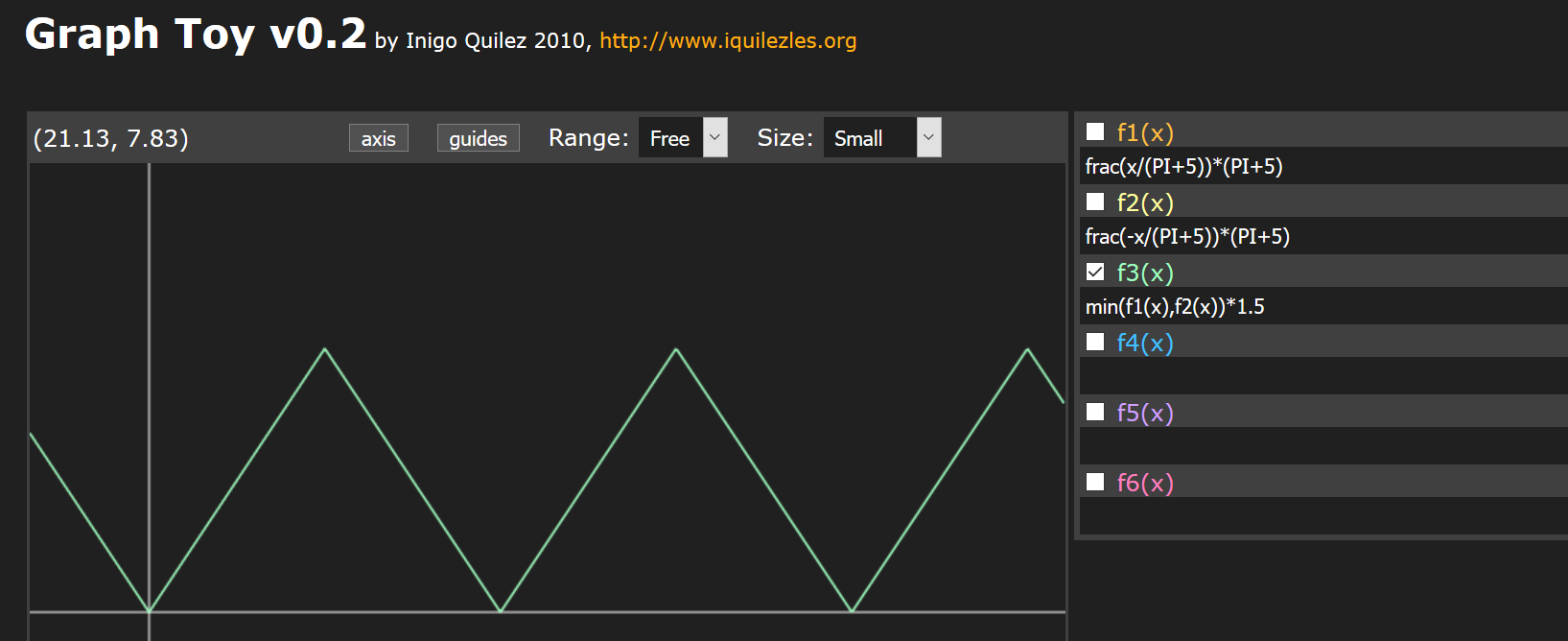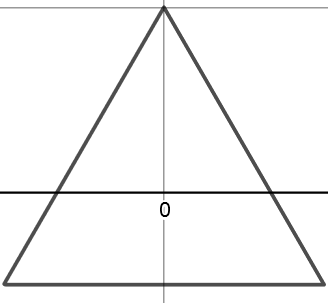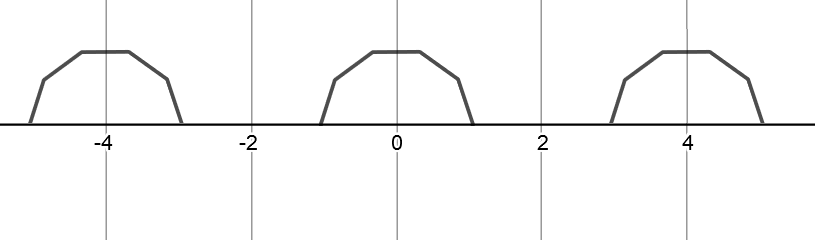# How can I draw regular polygons along the horizontal axis?

I drew simple triangle wave (that you can see It on graph toy).f1(x) = frac(x/(PI+5))*(PI+5)
f2(x) = frac(-x/(PI+5))*(PI+5)
f3(x) = min(f1(x),f2(x))*1.5


I want to change above shape like Approximating Pi and repeat It periodically along the horizontal axis.then I want to cut negative parts of the vertical axis:# Update

Note that I know how can I draw It by shader In ShaderToy.I Just need drawing mathematics In desmos or graphtoy,because I want Extract values of wave.I want to create polygon wave form.

I drew Periodic shape that I want to change the number of polygons Desoms Link.

• I'm not familiar with graphtoy, but I think I can explain how to do this in terms of raw math - would that help? – Pikalek Mar 13 '18 at 13:54
• @Pikalek Anyway, I'd appreciate if you answer my question – Seyed Morteza Kamali Mar 13 '18 at 14:33

You can use the equation of a circle to do this. A circle can be drawn using the equation:

$r^2 = x^2 + y^2$

You can solve for the upper half of this by rewriting it in terms of $y$ like this:

$y^2 = r^2 - x^2$

so

$y = ±√(r^2 - x^2)$

But we only want the positive half. So we say just:

$y = √(r^2 - x^2)$

You can then repeat it by using (((x - 2) % 4) - 2) as the input value. In the end the equation is this:

$y = √(1^2 - (((x - 2) mod 4) - 2)^2)$

It looks like this:In graph toy, it would be:

sqrt(1 - pow((((abs(x) - 2) % 4) - 2),2))

• I think the OP wants arbitrary regular polygons though, like in the Desmos they linked, not just circles. – Nathan Reed Mar 11 '18 at 2:14
• Instead of using sqrt I think max(sin(x),0) is better – Seyed Morteza Kamali Mar 12 '18 at 7:01
• @NathanReed that's right I need regular polygons not just circles – Seyed Morteza Kamali Mar 12 '18 at 7:39